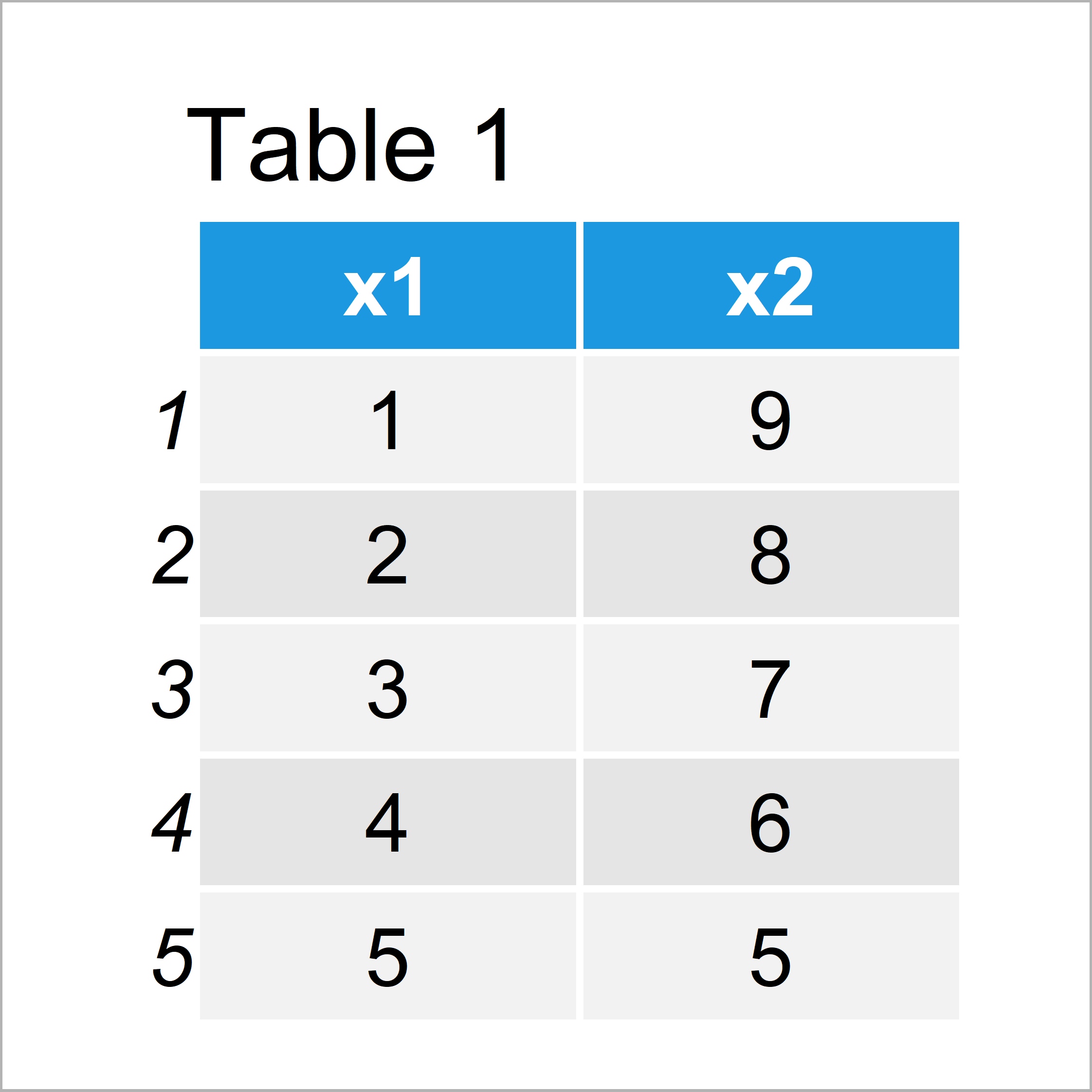# Warning Message in R: argument is not numeric or logical: returning NA

In this R tutorial you’ll learn how to handle the warning message: “argument is not numeric or logical: returning NA”.

So now the part you have been waiting for – the exemplifying R code…

## Creation of Example Data

As a first step, we’ll have to construct some data that we can use in the examples later on:

```data <- data.frame(x1 = 1:5,    # Create example data
x2 = 9:5)
data                            # Print example data```As you can see based on Table 1, our example data is a data frame and contains five rows and two variables.

## Example 1: Reproduce the Warning Message – argument is not numeric or logical: returning NA

The following R code shows how to replicate the warning message “argument is not numeric or logical: returning NA” in R.

Let’s assume that we want to know the mean of each column of our data frame. Then, we might try to apply the mean function as shown below:

```mean(data)                      # Apply mean function to data frame
#  NA
# Warning message:
#   In mean.default(data) : argument is not numeric or logical: returning NA```

The previous R code returns the warning message “argument is not numeric or logical: returning NA”. The reason for this is that the mean function cannot handle data frame objects as input.

So how can we solve this problem? That’s what I’ll explain next!

## Example 2: Fix the Warning Message – argument is not numeric or logical: returning NA

In Example 2, I’ll show how to avoid the warning “argument is not numeric or logical: returning NA”.

If we want to calculate the mean of all columns of a data frame, we have many different alternatives. One of them is based on the apply function:

```apply(data, 2, mean)            # Properly calculate mean of data frame columns
# x1 x2
#  3  7```

As you can see, the previous output returned a named vector that shows the mean of each variable of our data frame.

## Video & Further Resources

I have recently published a video on my YouTube channel, which illustrates the R syntax of the present tutorial. You can find the video below:

Furthermore, you may have a look at the other tutorials on my website. Some tutorials are shown here:

Summary: In this R tutorial you have learned how to deal with the warning “argument is not numeric or logical: returning NA”. If you have additional questions, please tell me about it in the comments.

Subscribe to the Statistics Globe Newsletter